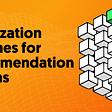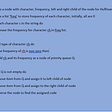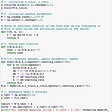# Stable Baselines Setup

I use tensorflow2.0, but stable-baselines use tensorflow1.x. So if you are using tf2, then use a virtual environment and install the dependencies.

`python3 -m venv venvsource venv/bin/activatepip install opencv-python==4.1.0.25pip install tensorflow==1.4pip install gympip install stable-baselines`

## Common errors

1. Stable Baselines do not support the new version of OpenCV (4.2). Hence it is one place where you may get cv2 import error, which can be solved by installing 4.1.0.25 version
2. Do not use tf2. Though you can manually use import `tensorflow.compat.v1 as tf` , by replacing all `import tensorflow as tf` in stable baselines package, which works fine for a few algorithms, fails for few. It raises `tensorflow.contrib.layers` doesn't have a `fully_connected` layer. This is deprecated in tf2. Hence use tensorflow1.x only.
3. Installing gym installs a minimal version of gym-envs. If you want to use atari and others, install `pip install gym[atari]` . In case you want all envs in the gym, use `pip install gym[all]`
4. For full version `brew install cmake openmpi` for mac users or `pip install stable-baselines[mpi]`. Stable baselines documentation suggests not to use the mpi version as it results in misbehavior in TensorFlow. If already done uninstall using `pip uninstall mpi4py`
5. Without mpi4py we cannot run DDPG, PPO1, and TRPO and hence not discussed in this post. If you want to see it running, for safe-side, I installed mpi4py in colab to see how the algorithms perform. We can also use another venv to install this and check without breaking anything.

# AC2

It has an actor and critic-networks where the actor updates the policy in the direction suggested by critic and critic estimates the value function.

The output of the actor can be stochastic [0.2, 0.4, 0.1, 0.3]-softmax output or deterministic [0,1,0,0] depending on the game requirement. `env.action_space.n` is the number of outputs that must be defined in the final layer of the actor-network. Input shape is the shape of the pixelated state which is called `obs` . Hence input shape is `envs.observation_space.shape` which is a tuple (num_inputs, height, width, channels).

The output of critic is policy_loss/actor loss.

• action = Actor(obs)
• Critic(action, rewards, mask) ==> Bellman equation
`for each frame:          for n_trials (update_interval):         # perform actor critic predictions -> pred == Estimates E()         # append value returned by critic to values         # append reward to returnscompute returns (gamma decay)     advantage = returns (list) - values (list)     calculate actor_loss(Jq)     calculate critic_loss(Jv)     calculate total_loss(J)     backpropagate(compute gradients) -> all updates = old+gradient`

Sometimes, the critic itself is called target network. Please don’t get confused.

Stable Baselines Code

`import gymfrom stable_baselines.common.policies import MlpPolicyfrom stable_baselines.common import make_vec_envfrom stable_baselines import A2Cenv = make_vec_env('CartPole-v1', n_envs=4)model = A2C(MlpPolicy, env, verbose=1)model.learn(total_timesteps=25000)model.save("a2c_cartpole")del modelmodel = A2C.load("a2c_cartpole")obs = env.reset()while True:    action, _states = model.predict(obs)    obs, rewards, dones, info = env.step(action)    env.render()`

# ACER

Actor-Critic with Experience Replay. Focussed on policy gradient variance reduction and to get an unbiased estimate; Retraces Q-value estimate; Applies clipped importance sampling ∏(target/behavior) on Q update. ACER uses Qret as the target to train the critic by minimizing the L2 error term: (Qret(s,a)−Q(s, a))2.

Uses efficient TRPO where instead of KL divergence, it uses a running average of past policies and forces the updated policy to not deviate far from this average.

`def compute_acer_loss(policies, q_values, values, actions, rewards, retrace, masks, behavior_policies, gamma=0.99, truncation_clip=10, entropy_weight=0.0001):    loss = 0        for step in reversed(range(len(rewards))):        importance_weight = policies[step].detach() / behavior_policies[step].detach()        retrace = rewards[step] + gamma * retrace * masks[step]        advantage = retrace - values[step]        log_policy_action = policies[step].gather(1, actions[step]).log()        truncated_importance_weight = importance_weight.gather(1, actions[step]).clamp(max=truncation_clip)        actor_loss = -(truncated_importance_weight * log_policy_action * advantage.detach()).mean(0)        correction_weight = (1 - truncation_clip / importance_weight).clamp(min=0)        actor_loss -= (correction_weight * policies[step].log() * (q_values[step] - values[step]).detach()).sum(1).mean(0)                entropy = entropy_weight * -(policies[step].log() * policies[step]).sum(1).mean(0)        q_value = q_values[step].gather(1, actions[step])        critic_loss = ((retrace - q_value) ** 2 / 2).mean(0)        truncated_rho = importance_weight.gather(1, actions[step]).clamp(max=1)        retrace = truncated_rho * (retrace - q_value.detach()) + values[step].detach()                loss += actor_loss + critic_loss - entropy            optimizer.zero_grad()    loss.backward()    optimizer.step()`

# ACKTR

Actor-Critic using Kronecker-factored Trust Region. This K-FAC is to do gradient update for both actor and critic.

# DDPG, D4PG

DDPG — Actions returned by the actor is not stochastic, it is deterministic. DDPG adds noise to action returned by the actor; Actions are normalized; Updates networks for every trial; do not compute returns for everything, select random minibatch and compute returns* for it (also called TD(λ)). Total loss is batch normalized; Make soft updates to actor and critic networks by θ = †θ + (1-†)θ’ where θ’ is actor and critic and θ is target_actor and target_critic.

• action = Actor(obs)
• Critic(action, rewards*, mask) ==> Bellman equation

Ornstein-Uhlenbeck process — Adding time-correlated noise to actions; evolve state to ou_state by x+dx where dx depends on sigma, then update sigma with time and decay_rate, return clipped(action + ou_state)

D4PG — Critic estimates Q value as random variable Zw; Qw(s,a)=𝔼Zw(x,a). Loss(J) = Bellman(Zw’, Zw) ie. same as DPG, but instead of ∆Q we use E(∆Q) where Q is the actor; Importance weight is calculated in actor update ie. Q update; Multiple Actors write to single replay buffer. Prioritized Experience Replay is in actor update.

# DQN

Replay buffer has state, action, reward, next_state, done. Target network is only periodically updated. Samples minibaches from replay buffer. Dueling DQN has a network to predict critic function and advantage function with shared network parameters.

# PPO2

Compute critic using TD(λ) estimator (minibatch returns) and Advantage with GAE(λ). Instead of normal advantage, we use

Generally, A = Q — V where Q = r + E(V); The above equation says, r is TD(λ) and n is lookahead. Not all V is added, only n lookaheads are considered. TD error is only for critic. Importance weight is multiplied to Advantage.

Clip the loss (for actor loss only) and add entropy term (for total loss). JCLIP(θ)=𝔼[min(r(θ)Â θold(s,a),clip(r(θ),1−ϵ,1+ϵ)Â θold(s,a))] JCLIP’(θ)=𝔼[JCLIP(θ)−c1(Vθ(s)−Vtarget)2+c2H(s,πθ(.))]

Updated after one update_interval; It is called PPO update and the loss is called PPO loss.

# SAC

SAC uses 1 Actor, 2 Critic networks (V valued and Q valued critics), and 1 target Critic Network thereby using the advantage of both Actor-Critic and DQN based solutions.

`class ReplayBuffer:    passclass NormalizedActions:    clip(actions, low, high)class ValueNetwork:  # Critic    passclass SoftQNetwork:  # Critic    # Critics can be Q value or V value    # Q value - Action-sate; V value - Value-state critics    passclass PolicyNetwork: # Actor    ...    ...    def evaluate(self, state, ε=1e-6):        mean, log_std = self.forward(state)        std = log_std.exp()        normal = Normal(mean, std)        z = normal.sample()        action = torch.tanh(z)        log_prob = normal.log_prob(z)-torch.log(1-action.pow(2)+ε)        log_prob = log_prob.sum(-1, keepdim=True)        return action, log_prob, z, mean, log_std    def get_action():        passdef soft_q_update(batch_size):   state,action,reward,next_s,done=replay_buffer.sample(batch_size)   expected_q_value = soft_q_net(state, action)   expected_value = value_net(state)   new_action,log_prob,z,mean,log_std = policy_net.evaluate(state)   target_value = target_value_net(next_state)   next_q_value = reward + (1 - done) * gamma * target_value   q_value_loss =           soft_q_criterion(expected_q_value,next_q_value.detach())   expected_new_q_value = soft_q_net(state, new_action)   next_value = expected_new_q_value - log_prob   value_loss = value_criterion(expected_value,next_value.detach())   log_prob_target = expected_new_q_value - expected_value   policy_loss=(log_prob*(log_prob-log_prob_target).detach()).mean()   mean_loss = mean_lambda * mean.pow(2).mean()   std_loss  = std_lambda  * log_std.pow(2).mean()   z_loss    = z_lambda    * z.pow(2).sum(1).mean()   policy_loss += mean_loss + std_loss + z_loss   # update soft_q_network   # update value_network   # update policy_network......value_net = ValueNetwork(state_dim, hidden_dim)target_value_net = ValueNetwork(state_dim, hidden_dim)soft_q_net = SoftQNetwork(state_dim, action_dim, hidden_dim)policy_net = PolicyNetwork(state_dim, action_dim, hidden_dim)for target_param, param in zip(target_value_net.parameters(),  value_net.parameters()):    target_param.data.copy_(param.data)value_criterion  = nn.MSELoss()soft_q_criterion = nn.MSELoss()value_optimizer  = optim.Adam(value_net.parameters(), lr=value_lr)soft_q_optimizer = optim.Adam(soft_q_net.parameters(), lr=soft_q_lr)policy_optimizer = optim.Adam(policy_net.parameters(), lr=policy_lr)......while frame_idx < max_frames:  ...  for step in range(max_steps):    ...    action = policy_net.get_action(state)    ...    replay_buffer.push(state, action, reward, next_state, done)    if len(replay_buffer) > batch_size:        soft_q_update(batch_size)    ...`

`from stable_baselines.common.vec_env import SubprocVecEnvfrom stable_baselines.common import set_global_seeds, make_vec_envnum_envs = 16                                    # 2*number of coresenv_name = "CartPole-v0"def make_env(env_name, rank, seed=0):    def _init():        env = gym.make(env_name)        env.seed(seed + rank)        return env    set_global_seeds(seed)    return _init...env = SubprocVecEnv([make_env(env_name, i) for i in range(num_cpu)])envs = SubprocVecEnv(envs)`

# Full code

• Here I have used only the MlpPolicy network (actor and critics). We can use any custom networks as policies.
`import gym, os, imageioimport numpy as npimport pandas as pdimport matplotlib.pyplot as pltfrom stable_baselines.common.policies import MlpPolicy as MlpCommon, MlpLstmPolicy, MlpLnLstmPolicyfrom stable_baselines.sac.policies import MlpPolicy as MlpSacfrom stable_baselines.td3.policies import MlpPolicy as MlpTD3from stable_baselines.deepq.policies import MlpPolicy as MlpDQNfrom stable_baselines.common.vec_env import DummyVecEnvfrom stable_baselines.common import set_global_seeds, make_vec_envfrom stable_baselines import A2C, ACER, ACKTR, DQN, PPO2, SAC, TD3from stable_baselines.common.vec_env import SubprocVecEnvfrom stable_baselines import results_plotterfrom stable_baselines.bench import Monitorfrom stable_baselines.results_plotter import load_results, ts2xyfrom stable_baselines.common.callbacks import BaseCallback, EvalCallbacklog_dir = "tmp/"os.makedirs(log_dir, exist_ok=True)class SaveOnBestTrainingRewardCallback(BaseCallback):    def __init__(self, check_freq: int, log_dir: str, verbose=1):        super(SaveOnBestTrainingRewardCallback, self).__init__(verbose)        self.check_freq = check_freq        self.log_dir = log_dir        self.save_path = os.path.join(log_dir, 'best_model')        self.best_mean_reward = -np.infdef _init_callback(self) -> None:        if self.save_path is not None:            os.makedirs(self.save_path, exist_ok=True)def _on_step(self) -> bool:        if self.n_calls % self.check_freq == 0:x, y = ts2xy(load_results(self.log_dir), 'timesteps')          if len(x) > 0:              mean_reward = np.mean(y[-100:])              if self.verbose > 0:                print("Num timesteps: {}".format(self.num_timesteps))                print("Best mean reward: {:.2f} - Last mean reward per episode: {:.2f}".format(self.best_mean_reward, mean_reward))if mean_reward > self.best_mean_reward:                  self.best_mean_reward = mean_reward                  if self.verbose > 0:                    print("Saving new best model to {}".format(self.save_path))                  self.model.save(self.save_path)return Truedef make_env(env_id, rank, seed=0):    def _init():        env = gym.make(env_id)        env.seed(seed + rank)        return env    set_global_seeds(seed)    return _initdef train_models(model,model_name,algorithm,environment,env=None):  global log_dir  if algorithm=='SAC':     callback = EvalCallback(           env,best_model_save_path='./tmp/sac_logs/',           log_path='./tmp/sac_logs/', eval_freq=100,            deterministic=True, render=False)  else:     callback = SaveOnBestTrainingRewardCallback(              check_freq=100,log_dir=log_dir)  time_steps = 100000   model.learn(total_timesteps=time_steps, log_interval=1, callback=callback)   model.save(os.path.join('tmp/models',model_name))results_plotter.plot_results([log_dir], time_steps, results_plotter.X_TIMESTEPS, model_name)  plt.savefig(os.path.join('tmp/results',model_name+'.png'))new_monitor_path = os.path.join('tmp/monitor_data',model_name+'.csv') with open('tmp/monitor.csv','r') as f:  with open(new_monitor_path,'w') as f1:   next(f)   for line in f:    f1.write(line)results = pd.read_csv(new_monitor_path) x = results['t'] y = results['r'] plt.plot(x,y) plt.savefig(os.path.join('tmp/results',model_name+'_curve.png'))images = [] obs = model.env.reset() img = model.env.render(mode='rgb_array') for _ in range(100):    images.append(img)    action, _states = model.predict(obs)    obs, _, _ ,_ = model.env.step(action)    img = model.env.render(mode='rgb_array') imageio.mimsave(os.path.join('tmp/results',model_name+'.gif'), [np.array(img) for i, img in enumerate(images) if i%2 == 0], fps=29)def train_env(environment):  global log_dir  env = gym.make(environment)  env = Monitor(env, log_dir)  param_noise = Nonea2c_model = A2C(MlpCommon, env, verbose=1)  acer_model = ACER(MlpCommon, env, verbose=1)  acktr_model = ACKTR(MlpCommon, env, verbose=1)  dqn_model = DQN(MlpDQN, env, prioritized_replay=True, verbose=1)  ppo2_model = PPO2(MlpCommon, env, verbose=1)  sac_model = SAC(MlpSac, environment)train_models(a2c_model, 'a2c_'+environment, 'A2C', environment)  train_models(acer_model, 'acer_'+environment, 'ACER', environment)  train_models(acktr_model, 'acktr_'+environment, 'ACKTR', environment)  train_models(dqn_model, 'dqn_'+environment, 'DQN',environment)  train_models(ppo2_model, 'ppo2_'+environment, 'PPO2',environment)  train_models(sac_model, 'sac_'+environment, 'SAC',environment,env)# # Classic Control# 'Acrobot-v1','MountainCar-v0','MountainCarContinuous-v0','CartPole-v1','Pendulum-v0'classic_contol_environments = ['CartPole-v1']  #?#?list(map(train_env, classic_contol_environments))# # Atariatari_environments = ['AirRaid-ram-v0','Alien-ram-v0','Boxing-ram-v0','Breakout-ram-v0','Freeway-ram-v0','IceHockey-ram-v0','Tennis-ram-v0']list(map(train_env, atari_environments))`

# Results over 1L episodes

## IceHockey

For equations and more details, this site has a good explanation.

--

--

--

## More from Amulya Reddy Konda

Consultant

Love podcasts or audiobooks? Learn on the go with our new app.

## Fighting Fraud — The Machine Learning Way## Bagging vs Boosting## Factorization Machines for Recommendation Systems## Classifying hotel reviews## Getting Started with TensorFlow: Basics of the TensorFlow API## Skew correction in Documents using Deep learning.## Billion-scale vector search with Vespa — part one## MLOps: Getting started with MlflowConsultant

## Deriving Mean Squared Error from Maximum Likelihood Estimation## Floyd’s cycle detection Algorithm## Huff man Coding## The Simplest Neural Network